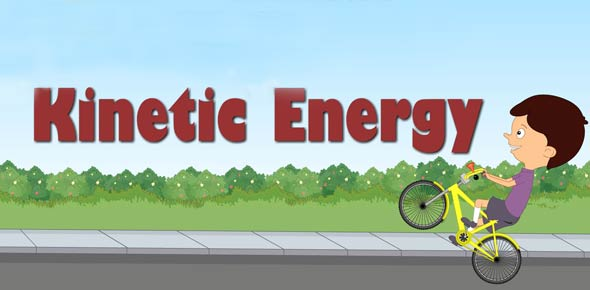# Kinetics Quiz #1

7 Questions | Total Attempts: 1234SettingsQuick quiz on reaction rates and enthalpys.

Related Topics
• 1.
The difference between the potential energy of the products and the potential energy of the reactants is the
• A.

Heat of fusion

• B.

Heat of reaction

• C.

Free energy

• D.

Activation energy

• E.

Positive energy

• 2.
When NH4NO3 is dissolved in water, the temperature of the water decreases.  When NaOH is dissolved in a separate water sample, the temperature of the water increases.  Based on these observations, it can be concluded that the dissolving of
• A.

Both salts are endothermic.

• B.

Both salts are exothermic.

• C.

NH4NO3 is exothermic and the dissolving of NaOH is endothermic.

• D.

NH4NO3 is endothermic and the dissolving of NaOH is exothermic.

• 3.
What is the heat of formation of 1 mole of H2O(g)?
• A.

-241.8 kj/mol

• B.

-483.6 kj/mol

• C.

-285.5 kj/mol

• D.

-571.6 kj/mol

• 4.
Activation energy is required to initiate
• A.

Endothermic reactions only.

• B.

Neither exothermic or endothermic reactions.

• C.

Both exothermic and endothermic reactions.

• D.

Exothermic reactions only.

• 5.
In the reversible reaction, the difference between the activation energy of the forward reaction and the activation energy of the reverse reaction is equal to the
• A.

Activated complex.

• B.

Potential energy of the products.

• C.

Potential energy of the reactants.

• D.

Heat of reaction.

• 6.
Which condition will increase the rate of a chemical reaction?
• A.

Increased temperature and decreased concentration of reactants

• B.

Increased temperature and increased concentration of reactants

• C.

Decreased temperature and decreased concentration of reactants

• D.

Decreased temperature and increased concentration of reactants.

• 7.
Given the reaction:     2 SO2(g) + O2(g) --> 2 SO3(g)  The rate of the forward reaction increases by adding more SO2 because the
• A.

Number of molecular collisions will increase

• B.

Temperature will increase

• C.

Forward reaction is endothermic

• D.

Reaction will shift to the left